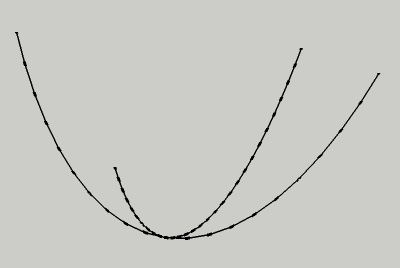Engineering ToolBox - Resources, Tools and Basic Information for Engineering and Design of Technical Applications!

# Solving Quadratic Equation with One Unknown

## How to solve a quadratic equation.

The quadratic equation can be expressed as

a x2 + b x + c = 0                      (1)

which can be solved for the two alternatives of x as

x1,2 = (-b ± (b2 - 4 a c)1/2) / (2 a)             (2)

### Example - Solving Quadratic Equation

If

a = 1

b = 6

c = 5

then the two alternatives for x can be calculated as

x1 = (-6 + (62 - 4 1 5)1/2) / (2 1)

= -1

x2 = (-6 - (62 - 4 1 5)1/2) / (2 1)

= -5## Related Topics

• ### Mathematics

Mathematical rules and laws - numbers, areas, volumes, exponents, trigonometric functions and more.

## Related Documents

• ### Algebraic Expressions

Principal algebraic expressions formulas.
• ### Circles within a Rectangle - Calculator

Calculate the maximum number of circles within a rectangle - can be used to calculate the numbers of pipes or wires in a conduit or similar.
• ### Discrete Data Sets and their Mean, Median and Mode Values

Calculate the arithmetic mean, median and modal values from discrete data sets.
• ### Logarithms

The rules of logarithms - log10 and loge for numbers ranging 1 to 1000.
• ### Numbers - Square, Cube, Square Root and Cubic Root Calculator

Calculate square, cube, square root and cubic root. Values tabulated for numbers ranging 1 to 100.
• ### Numbers - Standard Form, Scientific and Engineering Notation

The difference between standard, scientific and engineering number notation.
• ### Prime Numbers

Prime numbers up to 1000.
• ### Smaller Circles within a Large Circle - Calculator

Calculate the number of small circles that fits into an outer larger circle - ex. how many pipes or wires fits into a larger pipe or conduit.
• ### Solving Equations of the First Degree with One Unknown

Equations with one unknown.
• ### Solving First Degree Equations with Two Unknowns

Online calculator and solbing procedure for two equations with two unknowns.

## Engineering ToolBox - SketchUp Extension - Online 3D modeling!

Add standard and customized parametric components - like flange beams, lumbers, piping, stairs and more - to your Sketchup model with the Engineering ToolBox - SketchUp Extension - enabled for use with older versions of the amazing SketchUp Make and the newer "up to date" SketchUp Pro . Add the Engineering ToolBox extension to your SketchUp Make/Pro from the Extension Warehouse !

We don't collect information from our users. More about

## Citation

• The Engineering ToolBox (2022). Solving Quadratic Equation with One Unknown. [online] Available at: https://www.engineeringtoolbox.com/quadratic-equation-one-unknown-d_2209.html [Accessed Day Month Year].

Modify the access date according your visit.

9.19.12